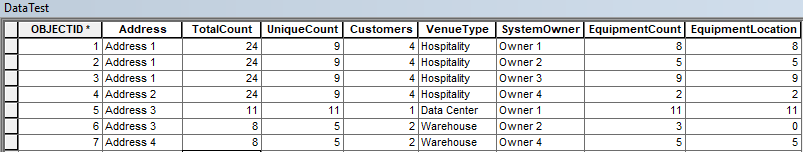# Excel SUMIFs using Python

5276
5
04-05-2019 01:23 PMOccasional Contributor II

Currently I'm using SUMIFs in Excel to calculate some values in a spreadsheet and then having to make the updates manually in my attribute tables or reloading all of my data into the feature class. Is there a way to do SUMIFs using Python in ArcMap? One method I came up with was using Summary Statistics. For my current workflow, I'd need to do it three different times and then either join the tables or use cursors to populate the information.

I need to get the sum of a field based on the address for another field's values. Another calculation is a max value based on address for another field, and then lastly need a count for an address for another field.My original thought process was to create a basic python tool using arcpy.Statistics_analysis (Summary Statistics) where my sum fields would be [[EquipmentCount, SUM], [EquipmentLocation, MAX], [Address, COUNT]] and my case field would be Address. The output would ideally be In Memory where I can use search cursor to build a dictionary where Address is my Key and the rest of the values would be the values. Using Update Cursor, I would populate the TotalCount field (using value of EquipmentCount - SUM), UniqueCount field (using EquipmentLocation - MAX), and Cutomers field (using Address - COUNT).

However I am trying to find another way to do this without having to go down the summary statistics route. I was hoping to use Field Calculator if possible or another alternative. I do not have any experience using the Pro-Logic in Field Calculators or numpy for that matter.

5 RepliesbyMVP Esteemed Contributor

Providing either sample or example data will allow commenters to give sample code in addition to workflow suggestions.

When you are finding the sum, max, and count for this address field; how are you storing the results?  Will the results be in a separate table with a single record for each address, or do you want to populate several records in the existing table with the same sum, max, and count values?Occasional Contributor II

Hi Joshua, I've updated my post with a screenshot of the table I have for testing.byMVP Legendary Contributor

yes, but you would have to get the data out to an appropriate format, but this demonstrates the principle

``````import numpy as np

names = np.asarray(['jim', 'sam', 'bill', 'sam'])
values = np.asarray([1, 2, 6, 5])
result = {name: values[names == name].sum() for name in np.unique(names)}

result
{'bill': 6, 'jim': 1, 'sam': 7}‍‍‍‍‍‍‍‍``````

Where

'names'  : is basically a column in a table

'values'   : is another column with the values you want to summarize

'result'  : in this case it is dictionary created by (a) getting the unique names in the names column, then for the values

column, it finds the matching entries for a particular name, then sums the value (in this example).  The dictionary is constructed from it but you could make a list and or array from it then create a table.

This missive was derived directly from the first hit on 'python sumif' in my browser

What format are your data?  2 columns? then it is simple... Do you just want a summary table? ditto

Any more details and/or data?Occasional Contributor II

Hi Dan, I've updated my post with a view of my table that I am using for testing with some further steps as to what I had in mind. My current method I can script out using python but I was hoping to find a faster method if possible.

I will try your way out in the mean time. Thank you!byMVP Legendary Contributor

``````# ---- some examples ---- see my blog for lots more

a = arcpy.da.TableToNumPyArray(in_fc, "*", skip_nulls=True)

a.dtype.names
('OBJECTID', 'Shape', 'Id', 'Long_1', 'Short_1', 'Float_1', 'Double_1', 'Text_1',
'DT_str', 'Shape_Length', 'Shape_Area')

a0 = a[['Long_1', 'Double_1', 'Text_1']]

a0

array([(1, 100., 'A 10 chars'), (3, 300., 'C not null')],
dtype={'names':['Long_1','Double_1','Text_1'], 'formats':['<i4','<f8','<U10'],
'offsets':[24,36,44], 'itemsize':180})

np.sum(a0['Long_1'])  # ---- some stats
4

np.min(a0['Double_1']) # ---- some stats
100.0

q = np.where(a0['Double_1'] > 200)  # ---- a little query

a0[q]   # ---- pull out the answer

array([(3, 300., 'C not null')],
dtype={'names':['Long_1','Double_1','Text_1'], 'formats':['<i4','<f8','<U10'], 'offsets':[24,36,44], 'itemsize':180})

# ---- and onward‍‍‍‍‍‍‍‍‍‍‍‍‍‍‍‍‍‍‍‍‍‍‍‍‍‍‍‍‍‍‍‍‍‍‍‍‍‍‍‍‍‍‍‍‍‍‍‍‍‍‍‍‍‍‍‍‍‍‍‍‍‍‍‍‍‍‍‍‍‍‍‍‍‍‍‍‍‍‍‍‍‍‍‍‍‍‍‍‍‍``````

``````# ---- instead of slicing use 'dot' notation by viewing the data as a record array

ar = a.view(np.recarray)

ar.Shape  # ---- pull up the geometry

array([[ 300005.  , 5000005.  ],
[ 300020.  , 5000010.22]])

ar.Long_1   # ---- ditto for another field
array([1, 3])

ar.DT_str    # ---- ditto, ditto
array(['2019/03/28 00:00:00', '2019/03/30 00:00:00'], dtype='<U20')
‍‍‍‍‍‍‍‍‍‍‍‍‍‍``````